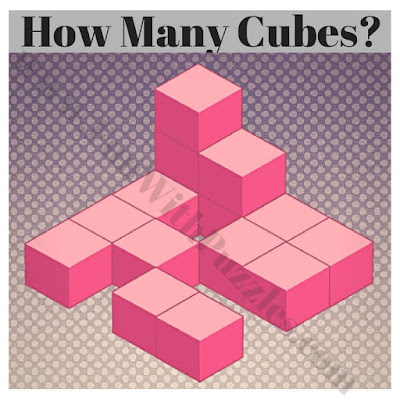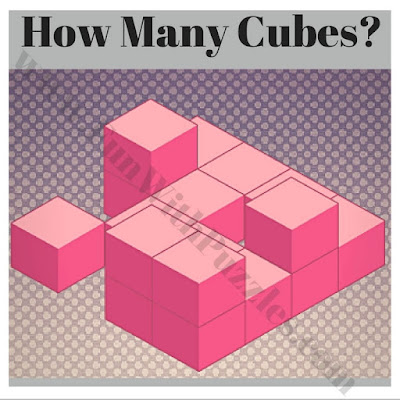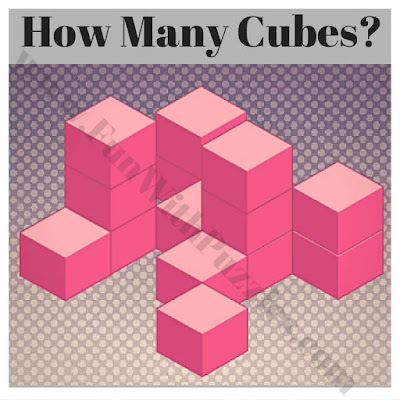Spatial Intelligence is the ability to understand three-dimensional figures and images. Logical Puzzles solving requires one's left side of the brain. However, solving spatial puzzles requires one's right side of the brain. Spatial puzzles do not require any knowledge of maths or language. However one needs to have very good observational skill to solve these kinds of puzzles. Here are some spatial puzzles in which one has to find the number of cubes in the given picture.
In these spatial puzzles, isometric views of cubes are given in each of the puzzles. One has to find the number of cubes which are present in the given figure puzzle.
Try to solve each puzzle as quick as possible and post your answers in the comment section. Answer of these spatial cube puzzles is given at the end. However, use these answers only to check your solutions.1. Picture puzzle to count cubes in the given figure2. Image puzzle to count cubes in figure3. Can you count the number of cube puzzle?4. How many cubes in picture puzzle?5. A spatial puzzle to count the number of cubes